# 深度学习（二十六）——VAE

https://antkillerfarm.github.io/

# VAE

《Auto-Encoding Variational Bayes》

https://kexue.fm/archives/5253

## 分布变换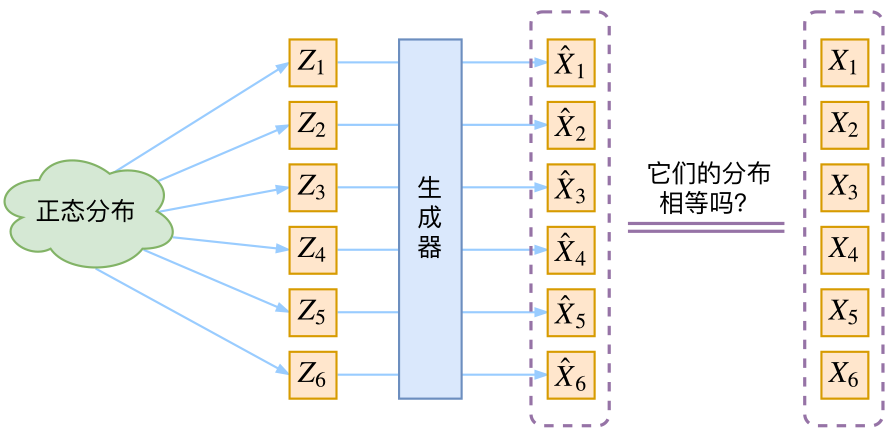## VAE的传统理解

$p(X)=\sum_Z p(X|Z)p(Z)$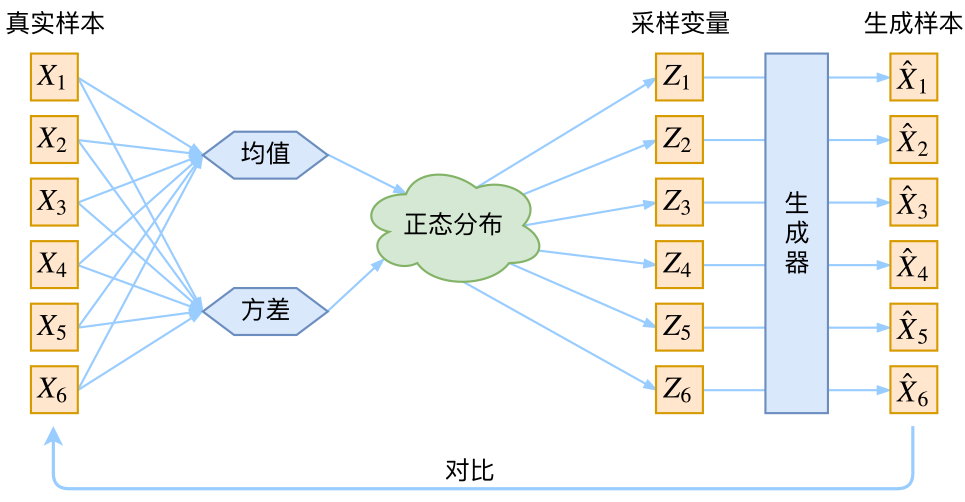## VAE初现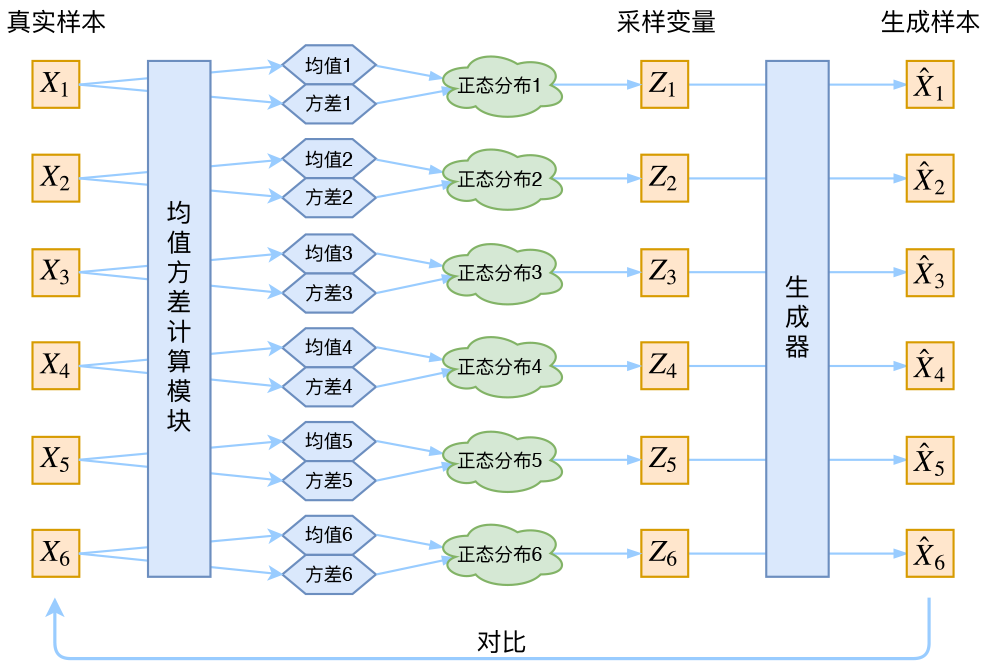## 分布标准化

$p(Z)=\sum_X p(Z|X)p(X)=\sum_X \mathcal{N}(0,I)p(X)=\mathcal{N}(0,I) \sum_X p(X) = \mathcal{N}(0,I)$

$\mathcal{L}_{\mu}=\Vert f_1(X_k)\Vert^2,\mathcal{L}_{\sigma^2}=\Vert f_2(X_k)\Vert^2$

$\mathcal{L}_{\mu,\sigma^2}=\frac{1}{2} \sum_{i=1}^d \Big(\mu_{(i)}^2 + \sigma_{(i)}^2 - \log \sigma_{(i)}^2 - 1\Big)$

\begin{aligned}&\mathcal{L}_{\mu,\sigma^2}=\mathcal{L}_{\mu} + \mathcal{L}_{\sigma^2}\\ &\mathcal{L}_{\mu}=\frac{1}{2} \sum_{i=1}^d \mu_{(i)}^2=\frac{1}{2}\Vert f_1(X)\Vert^2\\ &\mathcal{L}_{\sigma^2}=\frac{1}{2} \sum_{i=1}^d\Big(\sigma_{(i)}^2 - \log \sigma_{(i)}^2 - 1\Big)\end{aligned}

## Reparameterization Trick

$\mathcal{N}(\mu,\sigma^2)$中采样一个Z，相当于从$\mathcal{N}(0,I)$中采样一个$\varepsilon$，然后让$Z=\mu + \varepsilon \times \sigma$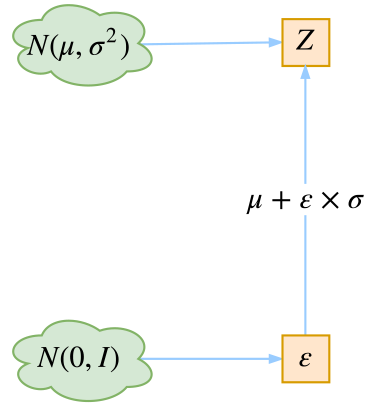## VAE本质

VAE本质上就是在我们常规的自编码器的基础上，对encoder的结果（在VAE中对应着计算均值的网络）加上了“高斯噪声”，使得结果decoder能够对噪声有鲁棒性；而那个额外的KL loss（目的是让均值为0，方差为1），事实上就是相当于对encoder的一个正则项，希望encoder出来的东西均有零均值。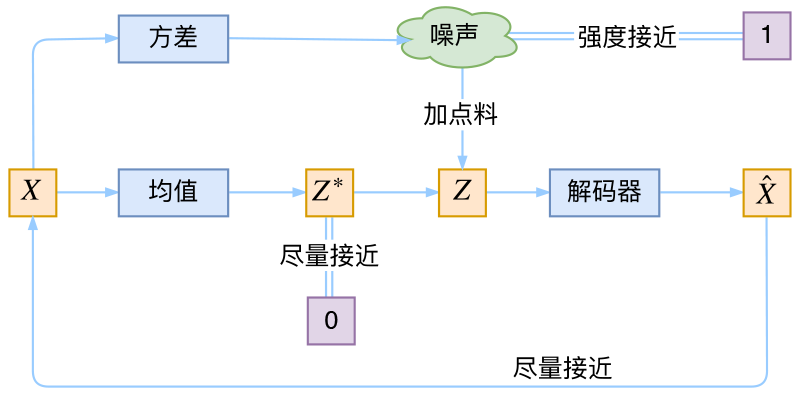## VAE vs AE

VAE和AE的差异在于：

1.两者虽然都是X->Z->X’的结构，但是AE寻找的是单值映射关系，即：$z=f(x)$

2.而VAE寻找的是分布的映射关系，即：$D_X\to D_Z$

VAE映射的是分布，而分布可以通过采样得到有效的z，从而生成相应的x。